Anúncio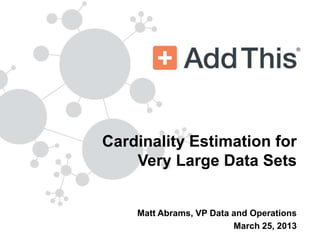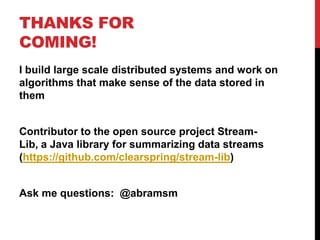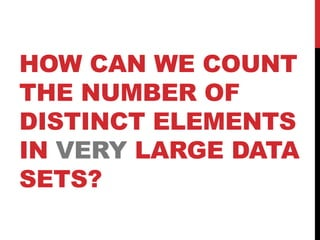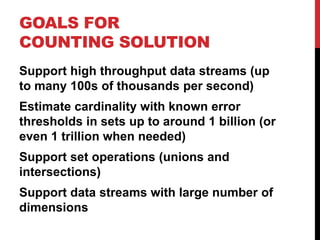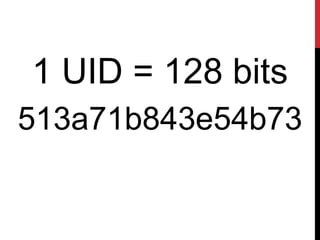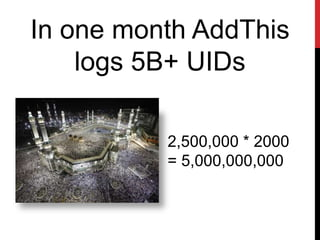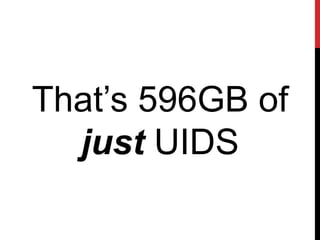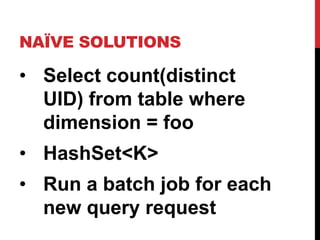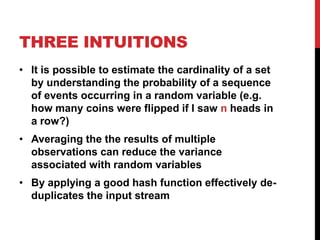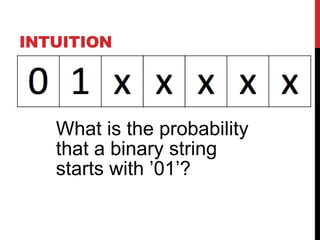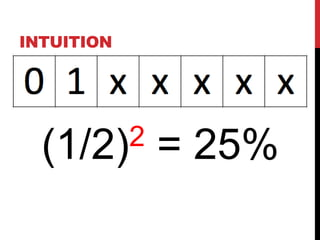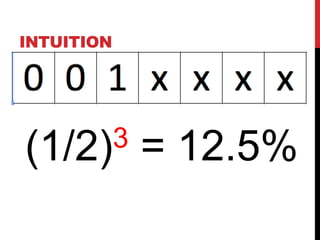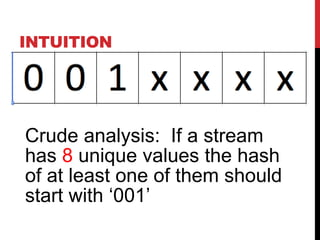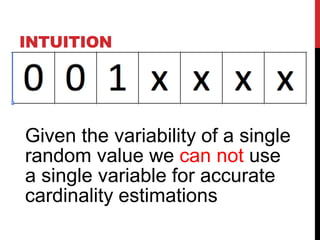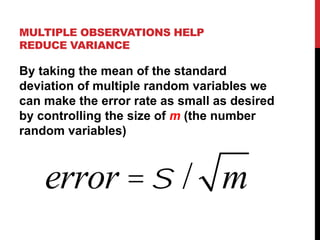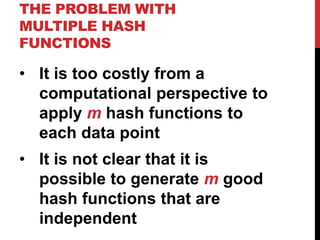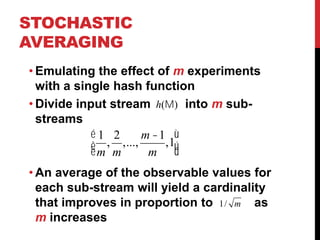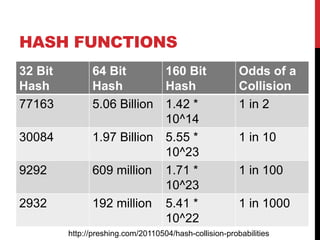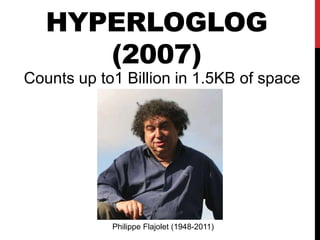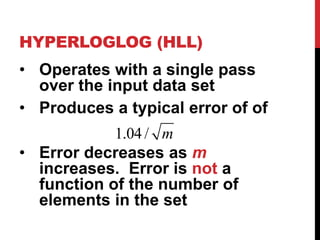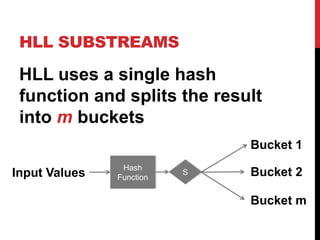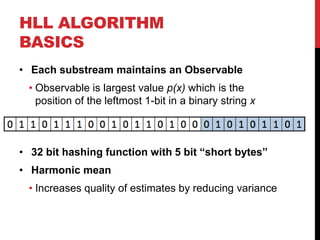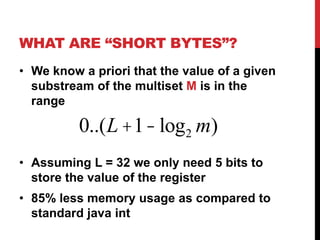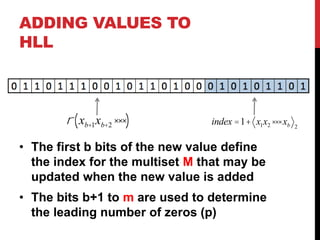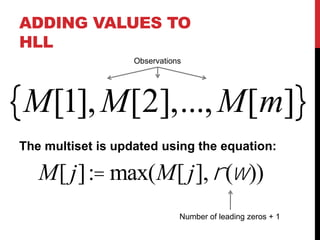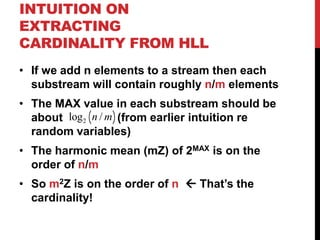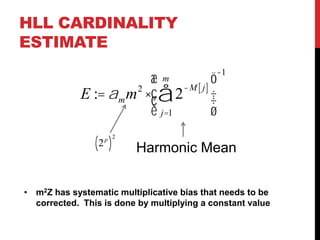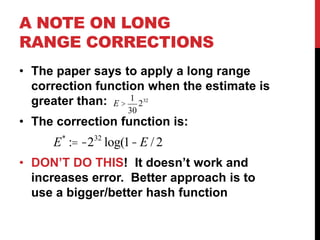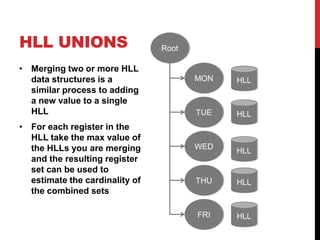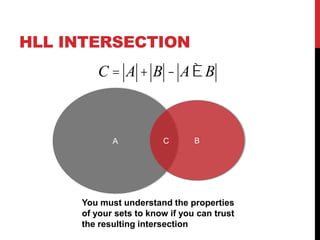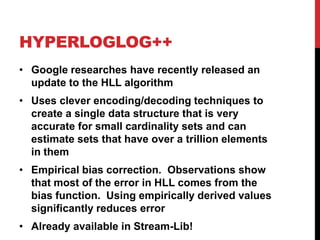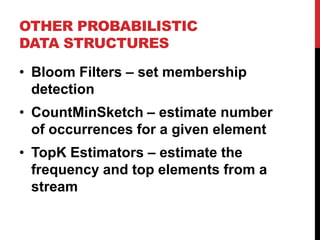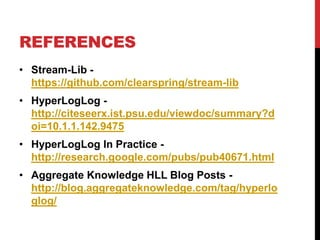1 de 40
Anúncio

### 2013 open analytics_countingv3

1. Cardinality Estimation for Very Large Data Sets Matt Abrams, VP Data and Operations March 25, 2013
2. THANKS FOR COMING! I build large scale distributed systems and work on algorithms that make sense of the data stored in them Contributor to the open source project Stream-Lib, a Java library for summarizing data streams (https://github.com/clearspring/stream-lib) Ask me questions: @abramsm
3. HOW CAN WE COUNT THE NUMBER OF DISTINCT ELEMENTS IN LARGE DATA SETS?
4. HOW CAN WE COUNT THE NUMBER OF DISTINCT ELEMENTS IN VERY LARGE DATA SETS?
5. GOALS FOR COUNTING SOLUTION Support high throughput data streams (up to many 100s of thousands per second) Estimate cardinality with known error thresholds in sets up to around 1 billion (or even 1 trillion when needed) Support set operations (unions and intersections) Support data streams with large number of dimensions
6. 1 UID = 128 bits 513a71b843e54b73
7. In one month AddThis logs 5B+ UIDs 2,500,000 * 2000 = 5,000,000,000
8. That’s 596GB of just UIDS
9. NAÏVE SOLUTIONS •  Select count(distinct UID) from table where dimension = foo •  HashSet<K> •  Run a batch job for each new query request
10. WE ARE NOT A BANK This means a estimate rather than exact value is acceptable. http://graphics8.nytimes.com/images/2008/01/30/timestopics/ feddc.jpg
11. THREE INTUITIONS •  It is possible to estimate the cardinality of a set by understanding the probability of a sequence of events occurring in a random variable (e.g. how many coins were flipped if I saw n heads in a row?) •  Averaging the the results of multiple observations can reduce the variance associated with random variables •  Applying a good hash function effectively de- duplicates the input stream
12. INTUITION What is the probability that a binary string starts with ’01’?
13. INTUITION (1/2)2 = 25%
14. INTUITION (1/2)3 = 12.5%
15. INTUITION Crude analysis: If a stream has 8 unique values the hash of at least one of them should start with ‘001’
16. INTUITION Given the variability of a single random value we can not use a single variable for accurate cardinality estimations
17. MULTIPLE OBSERVATIONS HELP REDUCE VARIANCE By taking the mean of the standard deviation of multiple random variables we can make the error rate as small as desired by controlling the size of m (the number random variables) error = σ / m
18. THE PROBLEM WITH MULTIPLE HASH FUNCTIONS •  It is too costly from a computational perspective to apply m hash functions to each data point •  It is not clear that it is possible to generate m good hash functions that are independent
19. STOCHASTIC AVERAGING • Emulating the effect of m experiments with a single hash function • Divide input stream h(Μ) into m sub- streams "1 2 m −1 % \$ , ,..., #m m ,1' m & • An average of the observable values for each sub-stream will yield a cardinality that improves in proportion to 1 / m as m increases
20. HASH FUNCTIONS 32 Bit 64 Bit 160 Bit Odds of a Hash Hash Hash Collision 77163 5.06 Billion 1.42 * 1 in 2 10^14 30084 1.97 Billion 5.55 * 1 in 10 10^23 9292 609 million 1.71 * 1 in 100 10^23 2932 192 million 5.41 * 1 in 1000 10^22 http://preshing.com/20110504/hash-collision-probabilities
21. HYPERLOGLOG (2007) Counts up to 1 Billion in 1.5KB of space Philippe Flajolet (1948-2011)
22. HYPERLOGLOG (HLL) •  Operates with a single pass over the input data set •  Produces a typical error of of 1.04 / m •  Error decreases as m increases. Error is not a function of the number of elements in the set
23. HLL SUBSTREAMS HLL uses a single hash function and splits the result into m buckets Bucket 1 Hash Input Values Function S Bucket 2 Bucket m
24. HLL ALGORITHM BASICS •  Each substream maintains an Observable •  Observable is largest value p(x) which is the position of the leftmost 1-bit in a binary string x •  32 bit hashing function with 5 bit “short bytes” •  Harmonic mean •  Increases quality of estimates by reducing variance
25. WHAT ARE “SHORT BYTES”? •  We know a priori that the value of a given substream of the multiset M is in the range 0..(L +1− log 2 m) •  Assuming L = 32 we only need 5 bits to store the value of the register •  85% less memory usage as compared to standard java int (32 bits)
26. ADDING VALUES TO HLL ρ ( xb+1 xb+2 ⋅⋅⋅) index = 1+ x1 x2 ⋅⋅⋅ xb 2 •  The first b bits of the new value define the index for the multiset M that may be updated when the new value is added •  The bits b+1 to m are used to determine the leading number of zeros (p)
27. ADDING VALUES TO HLL Observations {M, M,..., M[m]} The multiset is updated using the equation: M[ j] := max(M[ j], ρ (ω )) Number of leading zeros + 1
28. INTUITION ON EXTRACTING CARDINALITY FROM HLL •  If we add n unique elements to a stream then each substream will contain roughly n/m elements •  The MAX value in each substream should be about log 2 ( n / m) (from earlier intuition re random variables) •  The harmonic mean (mZ) of 2MAX is on the order of n/m •  So m2Z is on the order of n ß That’s the cardinality!
29. HLL CARDINALITY ESTIMATE −1 \$ m −M [ j ] ' E := α m m ⋅ & ∑ 2 2 & ) ) % j=1 ( p 2 (2 ) Harmonic Mean •  m2Z has systematic multiplicative bias that needs to be corrected. This is done by multiplying a constant value
30. A NOTE ON LONG RANGE CORRECTIONS •  The paper says to apply a long range correction function when the estimate is greater than: E > 1 232 30 •  The correction function is: E * := −2 32 log(1− E / 2 •  DON’T DO THIS! It doesn’t work and increases error. Better approach is to use a bigger/better hash function
31. DEMO TIME! Lets look at HLL in Action. http://www.aggregateknowledge.com/science/blog/hll.html
32. HLL UNIONS Root •  Merging two or more HLL data structures is a MON HLL similar process to adding a new value to a single HLL TUE HLL •  For each register in the HLL take the max value of the HLLs you are merging WED HLL and the resulting register set can be used to estimate the cardinality of THU HLL the combined sets FRI HLL
33. HLL INTERSECTION C = A + B − A∪B A C B You must understand the properties of your sets to know if you can trust the resulting intersection
34. HYPERLOGLOG++ •  Google researches have recently released an update to the HLL algorithm •  Uses clever encoding/decoding techniques to create a single data structure that is very accurate for small cardinality sets and can estimate sets that have over a trillion elements in them •  Empirical bias correction. Observations show that most of the error in HLL comes from the bias function. Using empirically derived values significantly reduces error
35. HLL++ DELTA ENCODING {1024,1027,1028,1030,1033,1035} {0, 3,1, 2, 3, 2} By using delta encoding fewer bits are required to represent array making it easier to fit larger sets in memory
36. OTHER PROBABILISTIC DATA STRUCTURES •  Bloom Filters – set membership detection •  CountMinSketch – estimate number of occurrences for a given element •  TopK Estimators – estimate the frequency and top elements from a stream
37. REFERENCES •  Stream-Lib - https://github.com/clearspring/stream-lib •  HyperLogLog - http://citeseerx.ist.psu.edu/viewdoc/summary? doi=10.1.1.142.9475 •  HyperLogLog In Practice - http://research.google.com/pubs/pub40671.html •  Aggregate Knowledge HLL Blog Posts - http://blog.aggregateknowledge.com/tag/ hyperloglog/